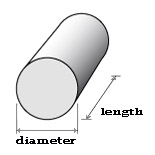# Metals Weight Calculator

Our online metals weight calculator enables you to calculate the weight of various materials with ease.

To use the calculator, follow these simple steps:

1. Choose the material type from the available options.
2. Select the shape and alloy of the metal.
3. Enter the relevant dimensions in the provided fields.
4. Input the number of pieces you want to calculate.
5. Click on the "Calculate" button to obtain the weight of the metal.

The metals weight calculator uses the basic equation below to calculate the metal weight:

Total Weight = # of Pieces * Volume * Density

Online Metals Weight Calculator
Material:
Alloy:
Shape:
Quantity (# of Pieces):inches feet yards mm cm m inches feet yards mm cm m inches feet yards mm cm m inches feet yards mm cm m

### Calculated Weights

Piece Weight (Lbs):

Total Weight (Lbs):

Total Weight (Kgs):